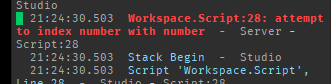# Table and dictionary problem

Hello, there I am making a system that requires that I need go find a specific part of a table.

Well let me explain

``````
local JOURS = {'Dimanche','Lundi','Mardi','Mercredi','Jeudi','Vendredi','Samedi'}

local CLASSE = {

Dimanche = {'congé'},

Lundi = {p1 = 'Français', p2 = 'Math', p3 = 'Science', p4 = 'Art plastique'},

Mardi = {p1 = 'Physique', p2 = 'Informatique', p3 = 'Math', p4 = 'Anglais'},

Mercredi = {p1 = 'Math', p2 = 'Français', p3 = 'Science', p4 = 'Éducation Physique'},

Jeudi = {p1 = 'Informatique', p2 = 'ECR', p3 = 'Art plastique', p4 = 'Physique'},

Vendredi = {p1 = 'Éducation Physique', p2 = 'Math', p3 = 'Français', p4 = 'Temps Libre'},

Samedi = {p1 = 'congé'},

}

number= 1

print(CLASSE[JOURS[numer]])
``````

so what I want to do is print the first thing of the Dimanche dictionary which would be ‘congé’

but when I’m doing that I get this error:how would I be able to print the string while using the ‘JOURS’ table to find the right one?

1 Like

I don’t really get what you mean. Could you specify more please?

From what I understood this is what you are searching for?

``````CLASSE[JOURS]
``````
2 Likes

wait was it that simple? Well yea that’s what I was trying to do lol and sorry if I wasn’t really clear

1 Like